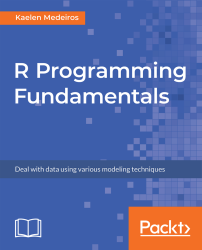•#### R Programming Fundamentals#### Overview of this book

R Programming Fundamentals, focused on R and the R ecosystem, introduces you to the tools for working with data. You’ll start by understanding how to set up R and RStudio, followed by exploring R packages, functions, data structures, control flow, and loops. Once you have grasped the basics, you’ll move on to studying data visualization and graphics. You’ll learn how to build statistical and advanced plots using the powerful ggplot2 library. In addition to this, you’ll discover data management concepts such as factoring, pivoting, aggregating, merging, and dealing with missing values. By the end of this book, you’ll have completed an entire data science project of your own for your portfolio or blog.
PrefaceFree Chapter
Introduction to RData Visualization and GraphicsData ManagementSolutionsOther Books You May Enjoy# Chapter 2: Data Visualization and Graphics

The following are the activity solutions for this chapter.

# Activity: Recreating Plots with Base Plot Methods

The following code can be used to load the datasets library:

`library(datasets)install.packages("ggplot2")library(ggplot2)`

The following code can be used to load the data:

`data("iris")data("mpg")`

The following code can be used to create a scatterplot to plot petal width without an axis label:

`plot(iris\$Petal.Width)`

The following code can be used to create a scatterplot to plot petal width without axis labels:

`plot(iris\$Petal.Width, iris\$Petal.Length,     main = "Petal Length vs. Petal Width",     xlab = "Petal Width",     ylab...`